# Some More Python Essentials

• 52k Accesses

Part of the Texts in Computational Science and Engineering book series (TCSE,volume 15)

## Abstract

We have seen that a group of numbers may be stored in an array that we may treat as a whole, or element by element. In Python, there is another way of organizing data that actually is much used, at least in non-numerical contexts, and that is a construction called list.

## 5.1 Lists and Tuples: Alternatives to Arrays

We have seen that a group of numbers may be stored in an array that we may treat as a whole, or element by element. In Python, there is another way of organizing data that actually is much used, at least in non-numerical contexts, and that is a construction called list.

### Some Properties of Lists

A list is quite similar to an array in many ways, but there are pros and cons to consider. For example, the number of elements in a list is allowed to change, whereas arrays have a fixed length that must be known at the time of memory allocation. Elements in a list can be of different type, so you may mix, e.g., integers, floats and strings, whereas elements in an array must be of the same type. In general, lists provide more flexibility than do arrays. On the other hand, arrays give faster computations than lists, making arrays our prime choice unless the flexibility of lists is needed. Arrays also require less memory use and there is a lot of ready-made code for various mathematical operations. Vectorization requires arrays to be used.

A list has elements that we may use for computations, just like we can with array elements. As with an array, we may find the number of elements in a list with the function len (i.e., we find the “length” of the list), and with the array function from numpy, we may create an array from an existing list: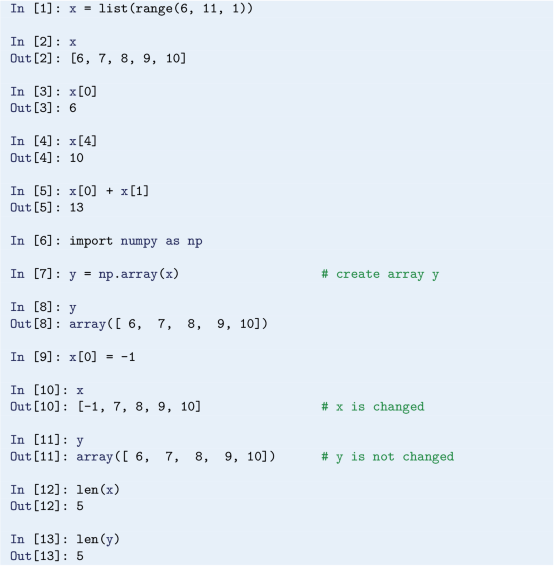A list may also be created by simply writing, e.g.,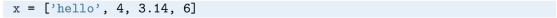giving a list where x contains the string hello, x contains the integer 4, etc.

We may add and delete elements anywhere in a list:Note the ways of writing the different operations here. Using append( ) will always increase the list at the end. If you like, you may create an empty list as x = [] before you enter a loop which appends element by element. Note that there are many more operations on lists possible than shown here.

### List and for Loops

Previously, we saw how a for loop may run over array elements. When we want to do the same with a list in Python, we may do it simply like: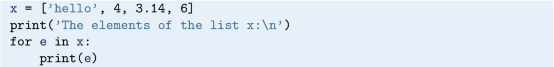We observe that e runs over the elements of x directly, avoiding the need for indexing. Be aware, however, that when loops are written like this, you can not change any element in x by “changing” e. That is, writing e += 2 will not change anything in x, since e can only be used to read (as opposed to overwrite) the list elements. Running the code gives the output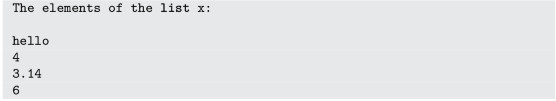### List Comprehension

There is a special construct in Python that allows you to run through all elements of a list, do the same operation on each, and store the new elements in another list. It is referred to as list comprehension and may be demonstrated as follows:So, we get a new list by the name L2, with the elements 10, 20, 30 and 40, in that order. Notice the syntax within the brackets for L2, e*10 for e in L1 signals that e is to successively be each of the list elements in L1, and for each e, create the next element in L2 by doing e*10. More generally, the syntax may be written aswhere E( e) means some expression involving e.

In some cases, it is required to run through 2 (or more) lists at the same time. Python has a handy function called zip for this purpose. An example of how to use zip is provided in Sect. 5.5 (file_handling.py).

### Some Properties of Tuples

We should also briefly mention about tuples, which are very much like lists, the main difference being that tuples cannot be changed. To a freshman, it may seem strange that such “constant lists” could ever be preferable over lists. However, the property of being constant is a good safeguard against unintentional changes. Also, it is quicker for Python to handle data in a tuple than in a list, which contributes to faster code. With the data from above, we may create a tuple and print the content by writing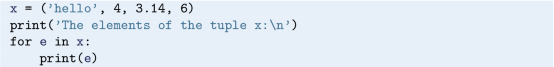Trying insert or append for the tuple gives an error message (because it cannot be changed), stating that the tuple object has no such attribute.

## 5.2 Exception Handling

An exception, is an error that is detected during program execution. We experienced such an error previously with our times tables program in Sect. 4.2.4. When we were asked about 3*2, and replied with the word six in stead of the number 6, we caused the program to stop and report about some ValueError. The program would have responded in the same way, for example, if we had rather given 6.0 (i.e., a float) as input, or just pressed enter (without typing anything else).

Our code could only handle “expected” input from the user, i.e., an integer as an answer to a*b. It would have been much better, however, if it could account also for “unexpected” input. If possible, we would prefer our program not to stop (or “crash”) unexpectedly for some kind of input, it should just handle it, get back on track, and keep on running. Can this be done in Python? Yes! Python has excellent constructions for dealing with exceptions in general, and we will show, in particular, how such exception handling will bring us to the fourth version of our times table program.

To get the basic idea with exception handling, we will first explain the very simplest try-except construction, and also see how it could be used in the times tables program. It will only partly solve our problem, so we will immediately move on to a more refined try-except construction that will be just what we need.

Generally, a simple try-except construction may be put up asWhen executed, Python recognizes the reserved words try and except (note colon and subsequent indent), and will do the following. First, Python will try to execute the statements in the try block. In the case when these statements execute without trouble, the except block is skipped (like the else block in an if-else construction). However, if something goes wrong in the try block, an exception is raised by Python, and execution jumps immediately to the except block without executing remaining statements of the try block.

It is up to the programmer what statements to have in the except block (as in the try block, of course), and that makes the programmer free to choose what will happen when an exception occurs! Sometimes, e.g., a program stop is desirable, sometimes not.

### Simple Use of try-except

Let us now make use of this simple try-except construction in the main program of times_tables_3.py, as a first attempt to improve the program. Doing so, the code may appear as (we give the whole program for easy reference):What will happen here? During execution, Python will first try to execute user_answer = ask_user(a, b) in the try block. If it executes without trouble, the except block is skipped, and execution continues with the line score = score + points(a, b, user_answer). However, if an exception is raised, execution proceeds immediately with print and continue in the except block. If so, the assignment to user_answer does not take place. The continue statement makes us move to the next question, since it immediately brings execution to the next loop iteration, i.e., score is neither updated, nor printed, before “leaving” that iteration.

This solution is a step forward for our program, since we avoid an unintentional stop if someone accidentally hits the wrong key. However, the except block here will handle all kinds of exceptions, and, in particular, trying to stop the program with Ctrl-c will no longer work (in stead, you may choose Consoles and Restart kernel from the Spyder menu)! It would be better programming to differentiate between different kinds of exceptions, coding a dedicated response in each case. That can be done in Python,Footnote 1 and it will also allow us to get back the Ctrl-c functionality that we had in the earlier versions.

### A More Detailed Use of try-except

We let the final version of our code (times_tables_4.py) serve as an example of a more refined try-except construction. It is still simple, but has what we need. We present this final version in its completeness, before explaining the details. All code changes are still confined to the main part of the program: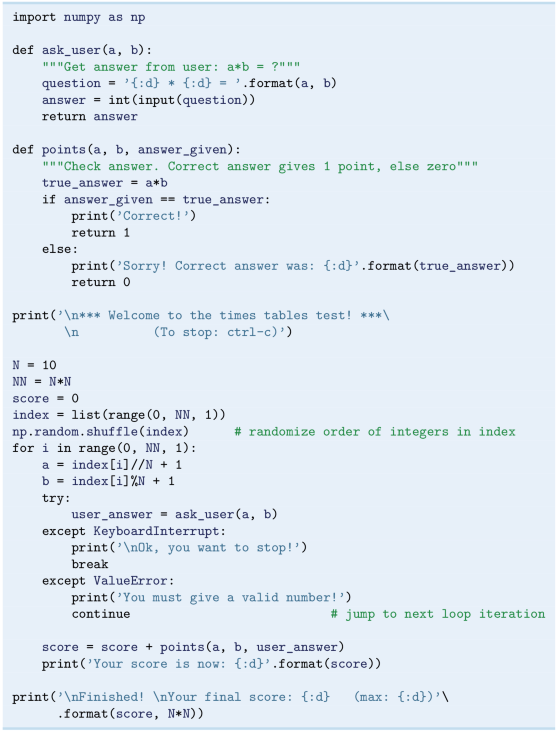Python has many different exception types, and we use two of them here, KeyboardInterrupt and ValueError (some more examples will be given soon). Unless the user answers with a valid integer, one of these exceptions is raised. A KeyboardInterrupt is raised if we type Ctrl-c to stop execution, whereas a ValueError is raised otherwise. We note that in each case an appropriate printout is given first. Furthermore, when a ValueError is raised, execution proceeds directly with the next question (after the printout). When a KeyboardInterrupt is raised, the printout is succeeded by execution of the break statement. This implies that execution breaks out of the for loop and the program stops after printing the final score.

One dialogue with the program could then be, for example: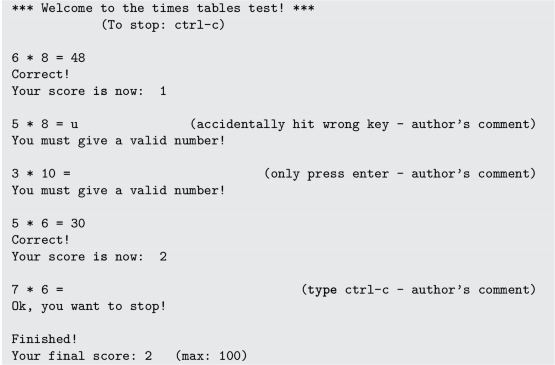With our final version, we see that some typical error situations are handled according to plan, and also that Ctrl-c now works as previously. For the present problem, we found that only two different types of exceptions (KeyboardInterrupt and ValueError) were required. Had more exceptions been needed, we could just have extended the structure straight forwardly, withfor each of them. Note that it is possible to have a unified response to several exceptions, by just collecting the exception types in a parentheses and separating them with a comma. For example, with two such exceptions, they would appear on the formBefore ending this chapter on exception handling, it is appropriate to briefly exemplify a few more of the many built-in exceptions in Python.

If we try to use an uninitialized variable, a NameError exception is raised:When division by zero is attempted, it results in a ZeroDivisionError exception:Using illegal indices causes Python to raise an IndexError exception.: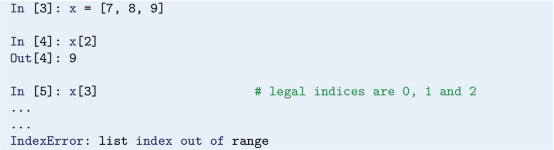Wrong Python grammar, or wrong typing of reserved words, gives a SyntaxError exception: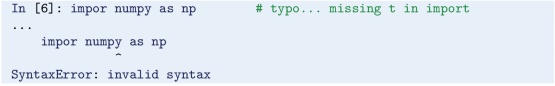If object types do not match, Python raises a TypeError exception: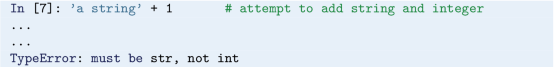(We might add that, in the last example here, two strings could have been straight forwardly concatenated with +.)

Abort Execution with sys.exit

In some cases, it is desirable to stop execution there and then. This may be done effectively by use of the exit function in the sys moduleFootnote 2 (a module with functions and parameters that are specific to the system). For an application of sys.exit, see Sect. 7.2.2.

We have been careful to check code behavior in a step-wise fashion while developing our program. Still, testing should be done also with (what, for now, is regarded as) the “final” version. To test our times tables program, we should check that all the 100 questions actually get asked, and also that points are given correctly. The simplicity of the present program allows this to be done while running it. Experienced programmers, however, usually write dedicated code for such testing. How to do this for implementations of numerical methods, will be presented later (see Chap. 6).

Note that, even if some error handling can be implemented by use of if-elif-else constructions, exception handling allows better programming, and is the preferred and modern way of handling errors. The recommendation to novice programmers is therefore to develop the habit of using try-except constructions.

## 5.3 Symbolic Computations

Even though the main focus in this book is programming of numerical methods, there are occasions where symbolic (also called exact or analytical) operations are useful.

### 5.3.1 Numerical Versus Symbolic Computations

Doing symbolic computations means, as the name suggests, that we do computations with the symbols themselves rather than with the numerical values they could represent. Let us illustrate the difference between symbolic and numerical computations with a little example. A numerical computation could be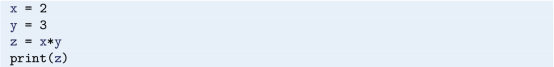which will make the number 6 appear on the screen.

A symbolic counterpart of this code could be written by use of the SymPy packageFootnote 3 (named sympy in Python):which causes the symbolic result x*y to appear on the screen. Note that no numerical value was assigned to any of the variables in the symbolic computation. Only the symbols were used, as when you do symbolic mathematics by hand on a piece of paper. Note also how symbol names must be declared by using symbols.

### 5.3.2 SymPy: Some Basic Functionality

The following script example_symbolic.py gives a quick demonstration of some of the basic symbolic operations that are supported in Python.Another useful possibility with sympy, is that sympy expressions may be converted to lambda functions, which then may be used as “normal” Python functions for numerical calculations. An example will illustrate.

Let us use sympy to analytically find the derivative of the function f(x) = 5x 3 + 2x 2 − 1, and then make both f and its derivative into Python functions:Note the arguments to lambdify. The first argument [x] specifies the argument that the generated function f (and the function dfdx) is supposed to take, while the second argument f_expr (and dfdx_expr) specifies the expression to be evaluated. When executed, the program prints 6 and 19, corresponding to f( 1) and dfdx( 1) , respectively.

Other symbolic calculations for, e.g., Taylor seriesFootnote 4 expansion, linear algebra (with matrix and vector operations), and (some) differential equation solving are also possible.

### 5.3.3 Symbolic Calculations with Some Other Tools

Symbolic computations are also readily accessible through the (partly) free online tool WolframAlpha,Footnote 5 which applies the very advanced Mathematica Footnote 6 package as symbolic engine. The disadvantage with WolframAlpha compared to the SymPy package is that the results cannot automatically be imported into your code and used for further analysis. On the other hand, WolframAlpha has the advantage that it displays many additional mathematical results related to the given problem. For example, if we type 2x + 3x - y in WolframAlpha, it not only simplifies the expression to 5x - y, but it also makes plots of the function f(x, y) = 5x − y, solves the equation 5x − y = 0, and calculates the integral $$\int \int (5x+y)dxdy$$. The commercial Pro version also offers a step-by-step demonstration of the analytical computations that solve the problem. You are encouraged to try out these commands in WolframAlpha:

• diff(xˆ2, x) or diff( x**2, x)

• integrate( cos( x) , x)

• simplify( ( x**2 + x**3) /x**2)

• limit( sin( x) /x, x, 0)

• solve( 5*x - 15, x)

WolframAlpha is very flexible with respect to syntax. In fact, WolframAlpha will use your input to guess what you want it to do! Depending on what you write, it may be more or less easy to do that guess, of course. However, in WolframAlpha’s response, you are also told how your input was interpreted, so that you may adjust your input in a second try.

Another impressive tool for symbolic computations is Sage,Footnote 7 which is a very comprehensive package with the aim of “creating a viable free open source alternative to Magma, Maple, Mathematica and Matlab”. Sage is implemented in Python. Projects with extensive symbolic computations will certainly benefit from exploring Sage.

## 5.4 Making Our Own Module

As we know by now, Python has a huge collection of useful modules and packages written by clever people. This far, we have experienced how these libraries (math, numpy, matplotlib, etc.) could simplify our own programming, making ready and professional code available through simple import statements. This is very good, but it gets even better, since it is straight forward (whether we are programming newbies or not) to also create modules containing our own code.

What is a module then, really? The truth is, that we may regard any of the Python scripts we have presented in this book as a module! In fact, any text file with extension .py that contains Python code written with a text editor, is a module. If the file name is my_module.py, then the module name is my_module. Up until now, we have written .py files for execution as programs. To design and use such files as module files, however, there are a few things we better get conscious about.

To bring across the essential points, we will develop our own little demonstration module for vertical motion, named vertical_motion (surprising!). The motion is of the kind we have addressed also previously in this book, i.e., a special case of projectile motion, in which an object starts out with some vertical velocity, and moves without any air resistance.Footnote 8 As with built-in modules, we will see that functionality may be imported in the usual ways, e.g., as import vertical_motion, which allows easy reuse of module functions.

### 5.4.1 A Naive Import

Before turning to the making of our vertical motion module, we will do some “warm-up” testing with a previous script of ours, just to enlighten ourselves a bit.

Let us pick ball_function.py from Sect. 4.1.1 (which addresses vertical motion), and argue that, if this script is a module, we should be able to “import it” as import ball_function, right? This sounds like a reasonable expectation, so without too deep reasoning, let us just start there.

First, however, we better take another look at that code (after all, it has been a while). For easy reference, we just repeat the few code lines of ball_function.py here:We recognize the function definition of y and the two applications of that function, involving a function call and a printout for each of the chosen points in time.

Now, we previously thought of this code as a program, executed it, and got the printouts. What will happen now, when we rather consider it a module and import it?

Here is what happens: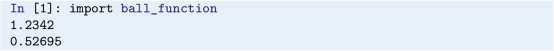What? Printing of numbers? We asked for an import,Footnote 9 not something that looks like program execution!

The thing is, that when any module is imported, Python does actually execute the module code (!), i.e., function definitions are read and statements (outside functions) executed. This is Python’s way of bringing module content “to life”, so that, e.g., functions defined in that module get ready for use. To see that the function y now is ready for use, we may proceed our interactive session as:Thus, apart from the undesirable printouts, the import seems to work!

To realize how inappropriate those printouts are, we might consider the following situation. A friend of yours wants to use your function y. You provide the file ball_function.py, your friend imports ball_function, and gets two numbers printed on the screen. Your friend did not ask for those numbers, and would probably end up reading your code to see what they were all about. It should not be like that.

Our main observation here, is that those undesirable printouts came from statements placed outside of functions. Thus, the lesson learned, is seemingly that when preparing modules for import, there should be no statements outside functions. Or, could there be a way to treat such statements, so that undesirable printouts are avoided? We will see.

These thoughts will be in the back of our minds as we now proceed to design the vertical motion module.

### Multiple Imports of the Same Module

Note that, when executing a program (or during an interactive session), Python does keep track of which modules that already have been imported. Thus, if another import is tried for a certain module, Python avoids the time consuming and unnecessary task of executing the module file once again (all module functionality is already in place, ready for use). You can check this out if you like, by doing a second import ball_function. This time, there are no printouts! Doing the import differently (i.e., with our example, as from ball_function import y or from ball_function import ∗), would not make any difference.

### 5.4.2 A Module for Vertical Motion

One simple way to avoid undesirable printouts during import, is to let the module file contain only function definitions. This is how we will arrange the first version of our vertical motion module.

We proceed to make ourselves a preliminary versionFootnote 10 of our new module file vertical_motion.py. In this file, we place three function definitions only (which should suffice for our demonstration). One of these, is the y function from ball_function.py, while the other two, time_of_flight and max_height, compute the time of flight and maximum height attained, respectively (consult any introductory book on mechanics regarding the implemented formulas).

In line with good programming practice, we also equip our module file with a doc string on top. Generally, that doc string should give the purpose of the module and explain how the module is used. More comprehensive doc strings are often required for larger and more “complicated” modules (here, our doc string is very simple, but professional programmers write their doc strings with great careFootnote 11). The module file reads:As with built-in modules, the built-in help function can be used to retrieve documentation from user-defined modules: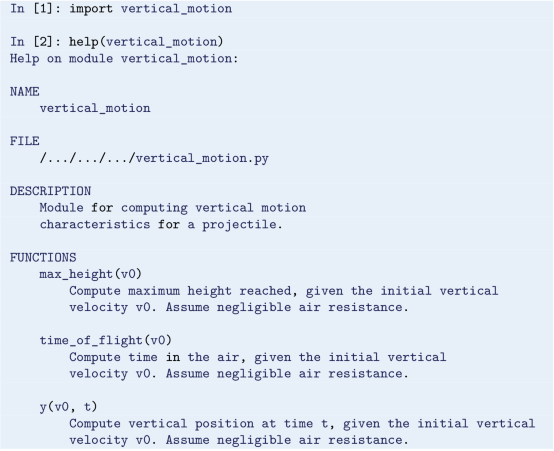We recognize the doc strings in the printout and should realize that it is a good idea to keep those doc strings informative.

With the following interactive session, comparing the answers to hand calculations (using the formulas and a calculator), we confirm that the module now seems to work as intended,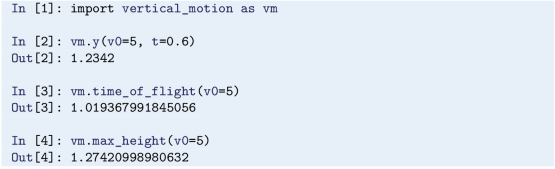We now have a useful version of our own vertical motion module, from which imports work just like from built-in modules. Still, there is room for useful modifications, as we will turn to next.

### Where to Place a Module File?

For a module import to be successful, a first requirement is that Python can find the module file. A simple way to make this happen, is to place the module file in the same folder as the program (that tries to import the module).12 There are other alternatives, but then you should know how Python looks for module files.

When Python proceeds to import a module, it looks for the module file within the folders contained in the sys.path list. To see the folders in sys.path, we may do: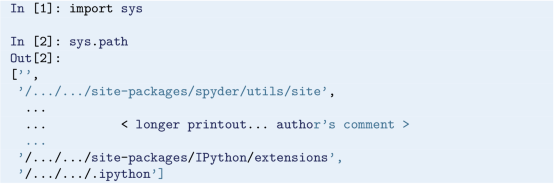Placing your module in one of the folders listed, assures that Python will find it. If, for some reason, you want to place your module in a folder that is not listed in sys.path, you may insert that folder name in sys.path. With our sys.path here, we could insert the folder name my_folder, for example, by continuing our session as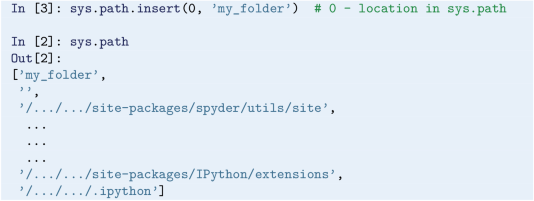TheFootnote 12 first argument to insert gives the location in the sys.path list where you want the folder name to be inserted (0 gives first place, 1 gives second, and so on).

You may also use the PYTHONPATH variable (https://docs.python.org/3/ using/cmdline.html#envvar-PYTHONPATH), but the above should suffice for a beginner.

If you want to run your module file also as a program, the location of the file might require that you first update the PATH environment variable (see, e.g., http://hplgit.github.io/primer.html/doc/pub/input/._input-readable009.html).

### 5.4.3 Module or Program?

We know how a .py file can be executed as a program, and we have seen how functions may be collected in a .py file, so that imports do not trigger any undesirable printouts. However, we have already realized that Python does not force a .py file to be either a program, or a module. No, it can be both, and thanks to a clever construction, Python allows a very flexible switch between the two ways of using a .py file.

This clever construction is based on an if test, which tests whether the file should be run as a program, or act as a module only. This is doable by use of the variable __name__, which (behind the scenes) Python sets to '__main__' only if the file is executed as a program (note the compulsory two underscores to each side of name and main here). We may put up a rather general form of the construction, that we place in the .py file, as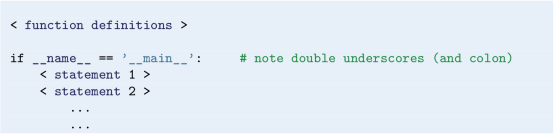So, if the file is run as a program, Python immediately sets __name__ to '__main__'. When reaching the if test, it will thus evaluate to True, which in turn causes the corresponding (indented) statements, i.e., the statements of the so-called test block, to be executed. To the contrary, if the file is used for imports only, __name__ will not be set to '__main__', the if test will consequently evaluate to False, and the corresponding statements are not executed.

Often, the statements in the test block are best placed in one or several functions (then defined above the if test, together with the other function definitions), so that when the if test evaluates to True, one or more function calls will follow. This is particularly important when different tasks are handled, so that each function contains statements that logically belong together.

As a simple illustration, when one function is natural (e.g., named application), the construction may be reformulated as### Our .py File as Both Module and Program

We will now incorporate this construction in vertical_motion.py. This allows us to use the functions from vertical_motion.py also in a program (our application) that asks the user for an object’s initial vertical velocity, and then computes height (as it develops with time), maximum height and flight duration.

The more flexible version of vertical_motion.py then reads,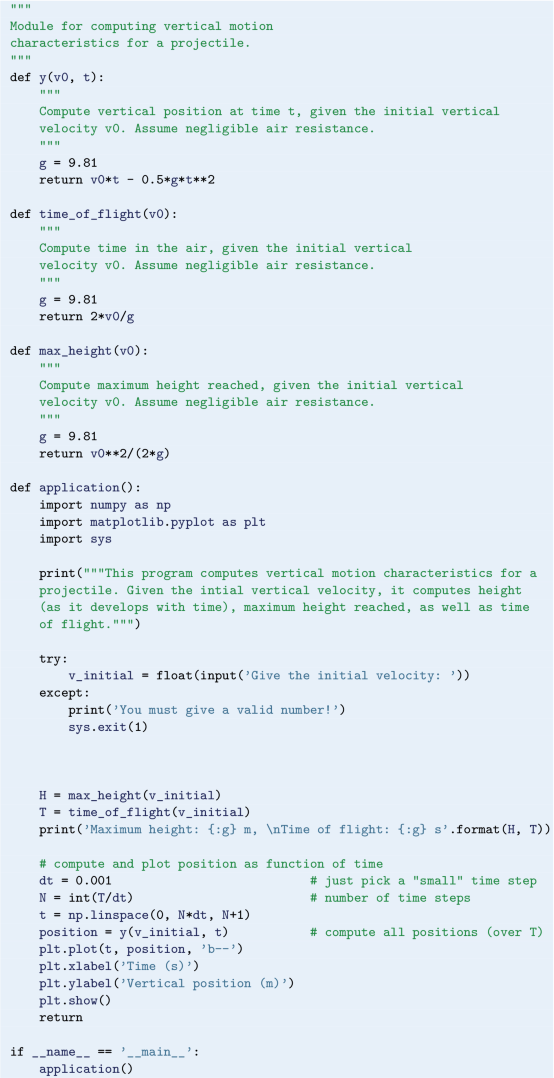The code in application represents the main program and should be understandable from what we have learned previously. Note that, like we have done here, it is usually a good idea to print some information about how the program works.

### Testing

As a simple test of the code in vertical_motion.py, we might compare the output to hand calculations, as we did before. In Chap. 6, however, we will learn how testing ought to be done via dedicated test functions. These test functions may be run in different ways. One alternative, however, is to include an option within the test block, which allows the user to run through the test functions whenever wanted, but more about that later.

Placing Import Statements in Our Module

Note that if we have import statements in our module, it is possible to run into trouble if we do not place them at the top of the file (which is according to the general recommendation).

With the following sketchy example module, it will work fine to import some_function in another program and use it (since, when importing some_function, the import of numpy is done).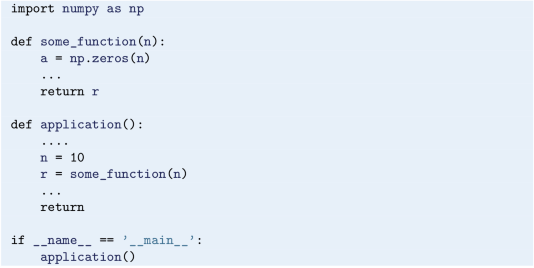One choice that would not work in the same way, however, would be to instead have the import statement import numpy as np after if __name__ == '__main__': . Then, this import statement would not be run if some_function is imported for use in another program.

## 5.5 Files: Read and Write

Input data for a program often come from files and the results of the computations are often written to file. To illustrate basic file handling, we consider an example where we read x and y coordinates from two columns in a file, apply a function f to the y coordinates, and write the results to a new two-column data file. The first line of the input file is a heading that we can just skip: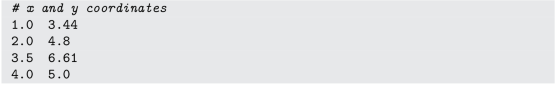The relevant Python lines for reading the numbers and writing out a similar file are given in the file file_handling.py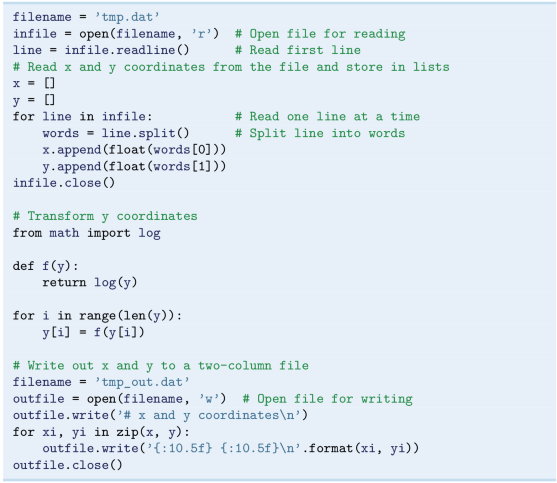If you have problems understanding the details here, make your own copy and insert printouts of line and the word elements in the (first) loop.

With zip, in the first iteration, xi and yi will represent the first element of x and y, respectively. In the second iteration, xi and yi will represent the second element of x and y, and so on.Footnote 13

## 5.6 Measuring Execution Time

Even though computational speed should have low priority among beginners to programming, it might be useful, at least, to have seen how execution time can be found for some code snippet. This is relevant for more experienced programmers, when it is required to find a particularly fast code alternative.

The measuring of execution time is complicated by the fact that a number of background processes (virus scans, check for new mail, check for software updates, etc.) will affect the timing. To some extent, it is possible to turn off such background processes, but that strategy soon gets too complicated for most of us. Fortunately, simpler and safer tools are available. To find the execution time of small code snippets, a good alternative is to use the timeit moduleFootnote 14 from the Python standard library.

### 5.6.1 The timeit Module

To demonstrate how this module may be used, we will investigate how function calls affect execution time. Our brief “investigation” is confined to the filling of an array with integers, done with and without a particular function call. The details are best explained with reference to the following code (no timing yet!):So, the sum of two integers is assigned to each array element. The arrays x and y will contain exactly the same numbers when the second loop is finished, but to fill x, we use a call to the function add. Thus, the time to fill x is expected to take longer than filling y, which just adds the numbers directly. Our question is, how much longer does it take to use the function call?

To answer this question by use of timeit, we may write the script timing_function_call.py: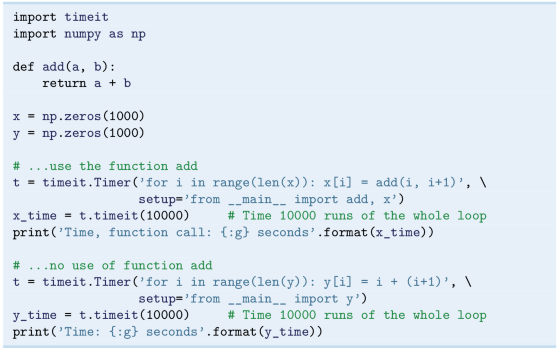What will happen here? Well, first of all, note that there are two calls to timeit.Timer, one for each of the two loops from above. If we look at the first call to timeit.Timer, i.e.,we notice that two arguments are provided. You may recognize the first argument, for i in range( len( x) ) : x[i] = add( i, i+1) , as a one-line version of the first loop from above, i.e. the loop over x (usually, we prefer to write such loops not on a single line. However, when used as an argument in a function call like here, the one-line version is handy). This first argument, given as a string, is what we want the timing of. The second argument, setup='from __main__ import add, x', is required for initialization, i.e., what the timer needs to do prior to timing of the loop. If you look carefully at the string-part of this second argument, you notice an import statement for add and x. You may wonder why you have to do that when they are defined in your code above, but stay relaxed about that, it is simply the way this timer function works. What is required for the timer function to execute the code given in the first argument, must be provided in the setup argument, even if it is defined in the code above.

The following line,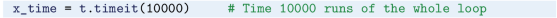will cause the whole loop to actually be executed, not a single time, but 10000 times! There will be one recorded time, the time required to run the loop 10000 times. Thus, if an average time for a single run-through of the loop is desired, we must divide the recorded time by (in this case) 10000. Often, however, the total time is fine for comparison between alternatives. The print command brings the recorded time to the screen, before the next loop is timed in an equivalent way.

Why is the loop run 10000 times? To get reliable timings, the execution times must be on the order of seconds, that is why. How many times the requested code snippet needs to be run, will of course depend on the code snippet in question. Sometimes, a single execution is enough. Other times, many more executions than 10000 are required. Some trial and error is usually required to find an appropriate number.

Executing the program produces the following result,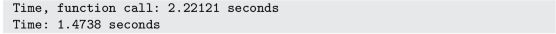So, using the function add to fill the array, takes 50% longer time!

## 5.7 Exercises

### Exercise 5.1: Nested for Loops and Lists

The code below has for loops that traverse lists of different kinds. One is with integers, one with real numbers and one with strings. Read the code and write down the printout you would have got if the program had been run (i.e., you are not supposed to actually run the program, just read it!).### Exercise 5.2: Exception Handling: Divisions in a Loop

Write a program that N times will ask the user for two real numbers a and b and print the result of a/b. Any exceptions should be handled properly (for example, just give an informative printout and let the program proceed with the next division, if any). The user should also be allowed to stop execution with Ctrl-c.

Set N = 4 in the code (for simplicity) and demonstrate that it handles different types of user input (i.e., floats, integers, text, just pressing enter, etc.) in a sensible way.

Filename: compute_division.py.

### Exercise 5.3: Taylor Series, sympy and Documentation

In this exercise, you are supposed to develop a Python function that approximates sin(x) when x is near zero. To do this, write a program that utilizes sympy to develop a Taylor series for sin(x) around x = 0, keeping only 5 terms from the resulting expression. Then, use sympy to turn the expression into a function. Let the program also plot sin(x) and the developed function together for x in [−π, π].

Filename: symbolic_Taylor.py.

### Remarks

Part of your task here, is to find and understand the required documentation. Most likely, this means that you have to seek more information than found in our book. You might have to read about the Taylor series (perhaps use Wikipedia or Google), and you probably have to look into more details about how Taylor series are handled with sympy.

To your comfort, this is a very typical situation for engineers and scientists. They need to solve a problem, but do not (yet!) have the required knowledge for all parts of the problem. Being able to find and understand the required information is then very important.

### Exercise 5.4: Fibonacci Numbers

The Fibonacci numbersFootnote 15 is a sequence of integers in which each number (except the two first ones) is given as a sum of the two preceding numbers: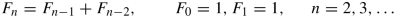Thus, the sequence starts out as

\displaystyle \begin{aligned} 1, 1, 2, 3, 5, 8, 13, 21, 34,\ldots\end{aligned}
1. a)

Write a function make_Fibonacci that generates, and returns, the N first Fibonacci numbers, when N is an input parameter to the function. Place the function in a module named fibonacci (i.e., a file named fibonacci.py). The module should have a test block, so that if run as a program, e.g., the 20 first Fibonacci numbers are printed to screen. Check that the program behaves as intended.

2. b)

The famous Johannes KeplerFootnote 16 found that the ratio of consecutive Fibonacci numbers converges to the golden ratio, i.e.

\displaystyle \begin{aligned} \lim_{n\rightarrow \infty}\frac{F_{n+1}}{F_n} = \frac{1+\sqrt{5}}{2}\, .\end{aligned}

Extend your module by defining a function converging_ratio, which takes an array (or a list) F with (e.g., 20) Fibonacci numbers as input and then checks (you decide how) whether Kepler’s understanding seems correct. Place a call to the function in the test block and run the program. Was Kepler right?

3. c)

With the iterative procedure of the previous question, the ratios converged to the golden ratio at a certain rate. This brings in the concept of convergence rate, which we have not yet addressed (see, e.g., Sect. 7.5, or elsewhere). However, if you are motivated, you may get a head start right now.

In brief, if we define the difference (in absolute value) between $$\frac {F_{n+1}}{F_n}$$ and the golden ratio as the error e n at iteration n, this error (when small enough) will develop as $$e_{n+1} = Ce_n^q$$, where C is some constant and q is the convergence rate (in fact, this error model is typical for iterative methods). That is, we have a relation that predicts how the error changes from one iteration to the next. We note that the larger the q, the quicker the error goes to zero as the number of iterations (n) grows (when e n < 1). With the given error model, we may compute the convergence rate from

\displaystyle \begin{aligned} q = \frac{\ln (e_{n+1}/e_n)}{\ln(e_n/e_{n-1})}\, .\end{aligned}

This is derived by considering the error model for three consecutive iterations, dividing one equation by the other and solving for q. If then a series of iterations is run, we can compute a sequence of values for q as the iteration counter n increases. As n increases, the computed q values are expected to approach the convergence rate that characterizes the particular iterative method. For the ratio we are looking at here, the convergence ratio is 1.

Extend your module with a function compute_rates, which takes an array (or a list) F with (e.g., 20) Fibonacci numbers as input and computes (and prints) the corresponding values for q. Call the function from the test block and run the program. Do the convergence rates approach the expected value?

Later, in Sect. 6.6.2, you will learn that convergence rates are very useful when testing (verifying) software.

Filename: Fibonacci_numbers.py.

### Exercise 5.5: Read File: Total Volume of Boxes

A file box_data.dat contains volume data for a collection of rectangular boxes. These boxes all have the same bottom surface area, but (typically) differ in height. The file could, for example, read:Apart from the header, each line represents one box. However, since they all have the same bottom surface area, that area (10.0) is only given for the first box. For that first box, also the height (3.0) is given, as it is for each of the following boxes.

1. a)

Write down a formula for computing the total volume of all boxes represented in the file. That formula should be written such that a minimum of multiplications and additions is used.

2. b)

Write a program that reads the file box_data.dat, computes the total volume of all boxes represented in the file, and prints that volume to the screen. In the calculations, apply the formula just derived.

(Note that, as a first step, you may read the file and just print (to screen) what is read. Comparing this printout with file content (use some editor) is then a good idea.)

3. c)

In the file box_data.dat, after the last line (containing the height of the “last” box), insert a couple of empty lines, i.e. just press enter a few times. Then, save the file and run the program anew. What happens? Explain briefly.

Filename: total_volume_boxes.py.

### Exercise 5.6: Area of a Polygon

One of the most important mathematical problems through all times has been to find the area of a polygon, especially because real estate areas often had the shape of polygons, and it was necessary to pay tax for the area. We have a polygon as depicted below.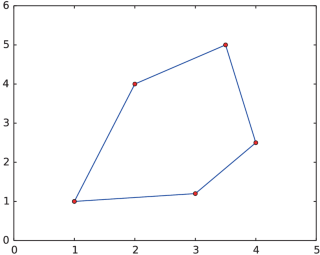The vertices (“corners”) of the polygon have coordinates (x 1, y 1), (x 2, y 2), …, (x n, y n), numbered either in a clockwise or counter clockwise fashion. The area A of the polygon can amazingly be computed by just knowing the boundary coordinates:

\displaystyle \begin{aligned} A \,{=}\, \frac{1}{2}\left| (x_1y_2+x_2y_3 + \cdots + x_{n-1}y_n + x_ny_1) - (y_1x_2 + y_2x_3 + \cdots + y_{n-1}x_n + y_nx_1)\right|\, .\end{aligned}

Write a function polyarea( x, y) that takes two coordinate arrays with the vertices as arguments and returns the area.

Test the function on a triangle, a quadrilateral, and a pentagon where you can calculate the area by alternative methods for comparison.

### Hint

Since Python lists and arrays have 0 as their first index, it is wise to rewrite the mathematical formula in terms of vertex coordinates numbered as x 0, x 1, …, x n−1 and y 0, y 1, …, y n−1.

Filename: polyarea.py.

### Exercise 5.7: Count Occurrences of a String in a String

In the analysis of genes one encounters many problem settings involving searching for certain combinations of letters in a long string. For example, we may have a string like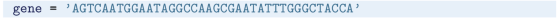We may traverse this string, letter by letter, by the for loop for letter in gene. The length of the string is given by len( gene) , so an alternative traversal over an index i is for i in range( len( gene) ) . Letter number i is reached through gene[i], and a substring from index i up to, but not including j, is created by gene[i:j].

1. a)

Write a function freq( letter, text) that returns the frequency of the letter letter in the string text, i.e., the number of occurrences of letter divided by the length of text. Call the function to determine the frequency of C and G in the gene string above. Compute the frequency by hand too.

2. b)

Write a function pairs( letter, text) that counts how many times a pair of the letter letter (e.g., GG) occurs within the string text. Use the function to determine how many times the pair AA appears in the string gene above. Perform a manual counting too to check the answer.

3. c)

Write a function mystruct( text) that counts the number of a certain structure in the string text. The structure is defined as G followed by A or T until a double GG. Perform a manual search for the structure too to control the computations by mystruct.

Filename: count_substrings.py.

### Remarks

You are supposed to solve the tasks using simple programming with loops and variables. While a) and b) are quite straightforward, c) quickly involves demanding logic. However, there are powerful tools available in Python that can solve the tasks efficiently in very compact code: a) text.count( letter) /len( text) ; b) text.count( letter*2) ; c) len( re.findall( ’G[AT]+?GG’, text) ) . That is, there is rich functionality for analysis of text in Python and this is particularly useful in analysis of gene sequences.

### Exercise 5.8: Compute Combinations of Sets

Consider an ID number consisting of two letters and three digits, e.g., RE198. How many different numbers can we have, and how can a program generate all these combinations?

If a collection of n things can have m 1 variations of the first thing, m 2 of the second and so on, the total number of variations of the collection equals m 1 m 2m n. In particular, the ID number exemplified above can have 26 ⋅ 26 ⋅ 10 ⋅ 10 ⋅ 10 = 676, 000 variations. To generate all the combinations, we must have five nested for loops. The first two run over all letters A, B, and so on to Z, while the next three run over all digits 0, 1, …, 9.

To convince yourself about this result, start out with an ID number on the form A3 where the first part can vary among A, B, and C, and the digit can be among 1, 2, or 3. We must start with A and combine it with 1, 2, and 3, then continue with B, combined with 1, 2, and 3, and finally combine C with 1, 2, and 3. A double for loop does the work.

1. a)

In a deck of cards, each card is a combination of a rank and a suit. There are 13 ranks: ace (A), 2, 3, 4, 5, 6, 7, 8, 9, 10, jack (J), queen (Q), king (K), and four suits: clubs (C), diamonds (D), hearts (H), and spades (S). A typical card may be D3. Write statements that generate a deck of cards, i.e., all the combinations CA, C2, C3, and so on to SK.

2. b)

A vehicle registration number is on the form DE562, where the letters vary from A to Z and the digits from 0 to 9. Write statements that compute all the possible registration numbers and stores them in a list.

3. c)

Generate all the combinations of throwing two dice (the number of eyes can vary from 1 to 6). Count how many combinations where the sum of the eyes equals 7.

Filename: combine_sets.py.

1. 1.
2. 2.
3. 3.

SymPy (http://docs.sympy.org/latest/index.html) is included in Anaconda. In case you have not installed Anaconda, you may have to install SymPy separately.

4. 4.

See, e.g., https://en.wikipedia.org/wiki/Taylor_series.

5. 5.
6. 6.
7. 7.
8. 8.

The reader who is into physics, will know that the computations done here, work equally well if the object has some simultaneous horizontal motion. In that case, our computations apply to the vertical component of the motion only, not the motion as a whole.

9. 9.

Doing the import differently, e.g., like from vertical_motion import y, would still give the printouts! Also if the import were done in a script.

10. 10.

Note that only the final version, presented in Sect. 5.4.3, is found on the book’s website.

11. 11.
12. 12.

a In this book, we keep to this simple way.***

13. 13.

Generally, zip allows running over multiple lists at the same time, ending when the shortest list is finished.

14. 14.
15. 15.

Read more about the Fibonacci numbers, e.g., on Wikipedia (https://en.wikipedia. org/wiki/Fibonacci_number).

16. 16.

Authors

## Rights and permissions

Open Access This chapter is licensed under the terms of the Creative Commons Attribution 4.0 International License (http://creativecommons.org/licenses/by/4.0/), which permits use, sharing, adaptation, distribution and reproduction in any medium or format, as long as you give appropriate credit to the original author(s) and the source, provide a link to the Creative Commons license and indicate if changes were made.

The images or other third party material in this chapter are included in the chapter's Creative Commons license, unless indicated otherwise in a credit line to the material. If material is not included in the chapter's Creative Commons license and your intended use is not permitted by statutory regulation or exceeds the permitted use, you will need to obtain permission directly from the copyright holder.

Reprints and Permissions